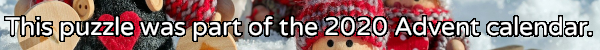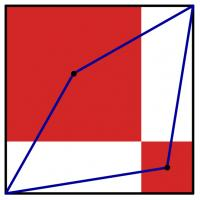mscroggs.co.uk
mscroggs.co.uksubscribe

# Puzzles

## 12 DecemberThe diagram to the left shows a large black square. Inside this square, two red squares have been drawn. (The sides of the red squares are parallel to the sides of the black square; each red square shares a vertex with the black square; and the two red squares share a vertex.) A blue quadrilateral has then been drawn with vertices at two corners of the black square and the centres of the red squares.
The area of the blue quadrilateral is 167. What is the area of the black square?

## 2 DecemberCarol draws a square with area 62. She then draws the smallest possible circle that this square is contained inside. Next, she draws the smallest possible square that her circle is contained inside. What is the area of her second square?

## 23 DecemberToday's number is the area of the largest area rectangle with perimeter 46 and whose sides are all integer length.

## 2 DecemberToday's number is the area of the largest dodecagon that it's possible to fit inside a circle with area $$\displaystyle\frac{172\pi}3$$.

## Squared circle

Each side of a square has a circle drawn on it as diameter. The square is also inscribed in a fifth circle as shown.
Find the ratio of the total area of the shaded crescents to the area of the square.

## Two triangles

Source: Maths Jam
The three sides of this triangle have been split into three equal parts and three lines have been added.
What is the area of the smaller blue triangle as a fraction of the area of the original large triangle?

## Overlapping triangles

Four congruent triangles are drawn in a square.
The total area which the triangles overlap (red) is equal to the area they don't cover (blue). What proportion of the area of the large square does each (purple) triangle take up?

## Unit octagon

The diagram shows a regular octagon with sides of length 1. The octagon is divided into regions by four diagonals. What is the difference between the area of the hatched region and the area of the region shaded grey?

## Archive

Show me a random puzzle
▼ show ▼
© Matthew Scroggs 2012–2021